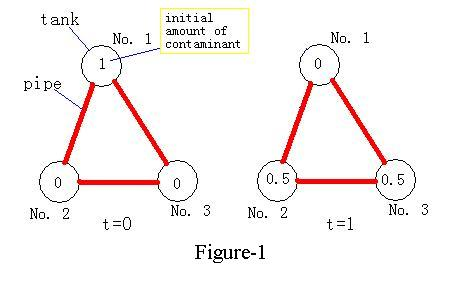Online JudgeProblem SetAuthorsOnline ContestsUser
Web Board
F.A.Qs
Statistical Charts
Problems
Submit Problem
Online Status
Prob.ID:
Register
Authors ranklist
Current Contest
Past Contests
Scheduled Contests
Award Contest
Register
Language:
Pollution
 Time Limit: 1000MS Memory Limit: 30000K Total Submissions: 4234 Accepted: 1140

Description

The managers of a chemical plant, which is notorious for its high pollution, plan to adopt a newly developed device in order to reduce the amount of contaminants emitted. However, engineers in the plant are against this plan, as they doubt the usefulness of the device. As engineers only believe in experimental results, managers decide to hire programmers to make a numerical experiment to convince the engineers.

The new pollution-reducing device consists of several tanks with pipes connecting the tanks. You may assume there is at most one pipe between two tanks. Two tanks are called adjacent if a pipe connects them. When operating, the contaminant circulates in the device among these tanks.As shown in the Figure-1, the contaminant in one tank in time t, will equally distribute into all adjacent tanks in the time t+1. In other words, if we use Xit to denote the amount of contaminant in tank i at time t, we can use the following formula:where Iij=1 if tank i and tank j are adjacent, otherwise Iij=0, and where dj is the number of tanks adjacent to tank j. If no tank is adjacent to tank i, we have Xit+1=Xit.
The managers, as well as the engineers, want to know that given the initial amount of contaminant in each tank, how the contaminant will be distributed in all the tanks after a long period of time in circulation. Namely, given Xi0 for all i, what are Xit when the difference between Xit and Xit+1 is so small that it can be ignored. You may assume that this condition will ALWAYS be attained from an initial case in this problem.

Input

The first line of the input contains one integer T (1 <= T <= 10), the number of test cases. T cases then follow. For each test case, the first line consists of two integers: N and M where(1 <= N <= 100, 0 <= M <= N*(N-1)/2), is the number of tanks and pipes. The following N lines give the initial amount of contaminant for each tank, which are nonnegative real numbers and no larger than 100. Then the next M lines give the tanks that each pipe connects, as "A B" (1 <= A, B <= N, A != B) denotes there is a pipe between tank A and tank B.

Output

For each test case, output the final amount of contaminant Xit+1 (one per line), followed by a blank line. The number should be rounded to three digits after the decimal point.

Sample Input

```2
3 3
1
0
0
1 2
2 3
3 1
4 4
1
0
0
1
1 2
2 3
3 1
3 4
```

Sample Output

```0.333
0.333
0.333

0.500
0.500
0.750
0.250
```

Source

[Submit]   [Go Back]   [Status]   [Discuss]Home PageGo BackTo top